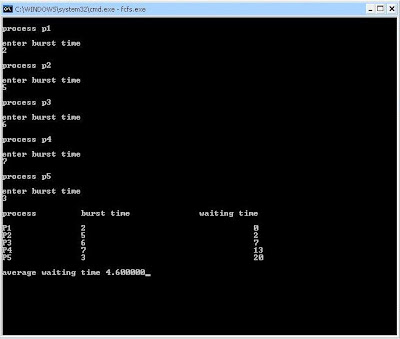### FCFS-CPU Scheduling in C

i was searching for source code for simulation of CPU Scheduling Algorithm FCFS in C on google
so i am going to post it here!

#include < stdio.h>
#include < conio.h>
struct process
{
int pid;
int burst;
int wait;
};
void main()
{
struct process p;
int i, j, wait=0;
float twait;
clrscr();
for(i=1;i<=5;i++)
{
p[i].pid=i;
printf("\nprocess p%d\n",i);
printf("\nenter burst time\n");
scanf("%d",&p[i].burst);
}
printf("\nprocess\t\tburst time\t\twaiting time\n");
for(i=1;i<=5;i++)
{
p[i].pid=i;
p[i].wait=wait;
printf("\nP%d\t\t%d\t\t\t\t %d",p[i].pid, p[i].burst,p[i].wait);
wait = wait + p[i].burst;
}
twait=wait;
printf("\n\naverage waiting time= %f",(twait/5));
getch();
}

Output for this code looks like this